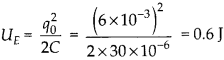Enlightened

# Suppose the initial charge on the capacitor given in question 7 is 6 mC. What is the total energy stored in the circuit initially? What is the total energy at later time?

• 0

Suppose the initial charge on the capacitor given in question 7 is 6 mC. What is the total energy stored in the circuit initially? What is the total energy at later time?

Share